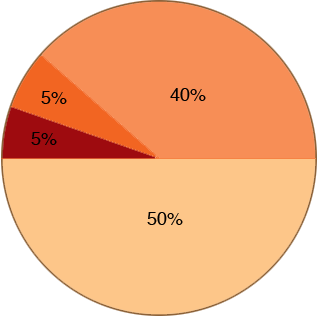SEARCH HOMEMath Central Quandaries & QueriesQuestion from Libby, a student: I don't understand how to do this word problem: The cafeteria workers distributed a survey to the student body asking students to pick 1 from a list of 5 choices for their favorite lunch. The circle graph below gives the results of the survey for the students who responded. What is the measure, in degrees, of the central angle for each choice? And there is a pie chart that has food choices with these percentages: 25%, 20%, 15%, 10%, and 30%We have two responses for you

Hi Libby,

Every circle has 360 degrees. Each of the central angles for your food choices can be found by calculating that percentage of the circle. For example, if the central angle was 5%, then the angle would be 5% of 360 degrees, or 0.05 x 360 = 18 degrees.

Hope this helps.
Leeanne

Hi Libby,

I am going to look at a circle graph where the percentages are different.First notice that the four percentages I have add to 100% as is the case with the five percentages in your question. This means that the wedges in the circle graph cover the entire circle.

All the way around the circle is 360o and the 50% wedge goes half way around so the central angle for the 50% wedge is 50% of 360o which is 1/2 × 360o = 180o.

The 40% wedge uses 40% of 360o as its central angle and hence the angle is 40% of 360o or 40/100 × 360 = 144o. Likewise the central angle for each of the two remaining wedges is 5% of 360o or 18o.

I hope this helps,
PennyMath Central is supported by the University of Regina and The Pacific Institute for the Mathematical Sciences.